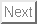Up: Sample Output Previous: Sample Output for n   Contents

## Sample Output for n = 33

```Welcome to the simulation of Shor's algorithm.
There are four restrictions for Shor's algorithm:
1) The number to be factored (n) must be >= 15.
2) The number to be factored must be odd.
3) The number must not be prime.
4) The number must not be a prime power.

There are efficient classical methods of factoring any of the above numbers, or determining that they are prime.

Input the number you wish to factor.
33
Step 1 starting.
Step 1 complete.
Step 2 starting.
Searching for q, the smallest power of 2 greater than or equal to n^2.
Found q to be 2048.
Step 2 complete.
Step 3 starting.
Searching for x, a random integer coprime to n.
Found x to be 8.
Step 3 complete.
Step 4 starting.
Made register 1 with register size = 12
Created register 2 of size 6
Step 4 complete.
Step 5 starting attempt: 1
Step 5 complete.
Step 6 starting attempt: 1
Step 6 complete.
Step 7 starting attempt: 1
Step 7 complete.
Step 8 starting attempt: 1
Making progress in Fourier transform, 4.83635% done!
Making progress in Fourier transform, 9.72154% done!
Making progress in Fourier transform, 14.6067% done!
Making progress in Fourier transform, 19.4919% done!
Making progress in Fourier transform, 24.3771% done!
Making progress in Fourier transform, 29.2623% done!
Making progress in Fourier transform, 34.1475% done!
Making progress in Fourier transform, 39.0327% done!
Making progress in Fourier transform, 43.9179% done!
Making progress in Fourier transform, 48.8031% done!
Making progress in Fourier transform, 53.6883% done!
Making progress in Fourier transform, 58.5735% done!
Making progress in Fourier transform, 63.4587% done!
Making progress in Fourier transform, 68.3439% done!
Making progress in Fourier transform, 73.2291% done!
Making progress in Fourier transform, 78.1143% done!
Making progress in Fourier transform, 82.9995% done!
Making progress in Fourier transform, 87.8847% done!
Making progress in Fourier transform, 92.7699% done!
Making progress in Fourier transform, 97.6551% done!
Step 8 complete.
Step 9 starting attempt: 1
Value of m measured as: 409
Step 9 complete.
Steps 10 and 11 starting attempt: 1
Measured m: 409, rational approximation for m/q=0.199707 is: 273 / 1367
Odd period found.  This trial failed. 	 Trying again.
Steps 10 and 11 complete.
Step 5 starting attempt: 2
Step 5 complete.
Step 6 starting attempt: 2
Step 6 complete.
Step 7 starting attempt: 2
Step 7 complete.
Step 8 starting attempt: 2
Making progress in Fourier transform, 4.83635% done!
Making progress in Fourier transform, 9.72154% done!
Making progress in Fourier transform, 14.6067% done!
Making progress in Fourier transform, 19.4919% done!
Making progress in Fourier transform, 24.3771% done!
Making progress in Fourier transform, 29.2623% done!
Making progress in Fourier transform, 34.1475% done!
Making progress in Fourier transform, 39.0327% done!
Making progress in Fourier transform, 43.9179% done!
Making progress in Fourier transform, 48.8031% done!
Making progress in Fourier transform, 53.6883% done!
Making progress in Fourier transform, 58.5735% done!
Making progress in Fourier transform, 63.4587% done!
Making progress in Fourier transform, 68.3439% done!
Making progress in Fourier transform, 73.2291% done!
Making progress in Fourier transform, 78.1143% done!
Making progress in Fourier transform, 82.9995% done!
Making progress in Fourier transform, 87.8847% done!
Making progress in Fourier transform, 92.7699% done!
Making progress in Fourier transform, 97.6551% done!
Step 8 complete.
Step 9 starting attempt: 2
Value of m measured as: 1438
Step 9 complete.
Steps 10 and 11 starting attempt: 2
Measured m: 1438, rational approximation for m/q=0.702148 is: 719 / 1024
Candidate period is 1024
8^512 + 1 mod 33 = 32,
8^512 - 1 mod 33 = 30
33 = 3 * 11
Steps 10 and 11 complete.
```Up: Sample Output Previous: Sample Output for n   Contents
Matthew Hayward - Quantum Computing and Shor's Algorithm GitHub Repository